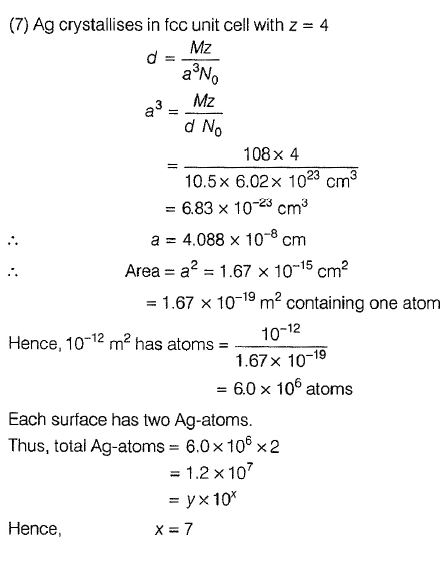Courses

# Revisal Problems (Past 13 Year) JEE Advanced

## 32 Questions MCQ Test Chemistry for JEE Advanced | Revisal Problems (Past 13 Year) JEE Advanced

Description
This mock test of Revisal Problems (Past 13 Year) JEE Advanced for JEE helps you for every JEE entrance exam. This contains 32 Multiple Choice Questions for JEE Revisal Problems (Past 13 Year) JEE Advanced (mcq) to study with solutions a complete question bank. The solved questions answers in this Revisal Problems (Past 13 Year) JEE Advanced quiz give you a good mix of easy questions and tough questions. JEE students definitely take this Revisal Problems (Past 13 Year) JEE Advanced exercise for a better result in the exam. You can find other Revisal Problems (Past 13 Year) JEE Advanced extra questions, long questions & short questions for JEE on EduRev as well by searching above.
QUESTION: 1

### Only One Option Correct Type This section contains 15 multiple choice questions. Each question has four choices (a), (b), (c) and (d), out of which ONLY ONE is correct.?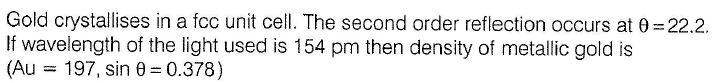Solution: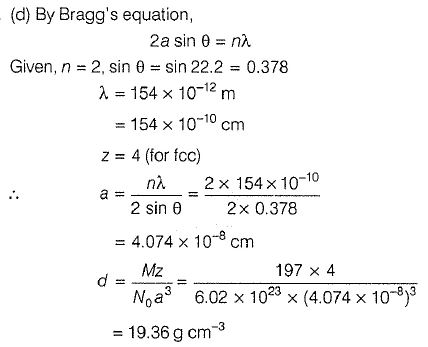QUESTION: 2

###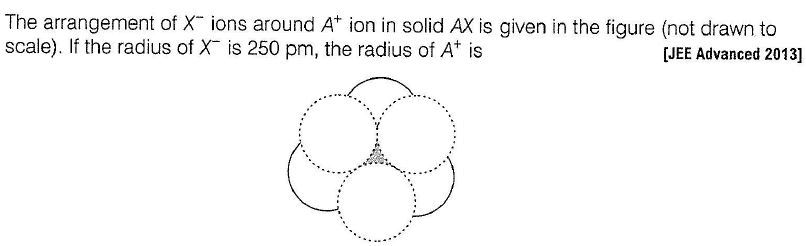Solution: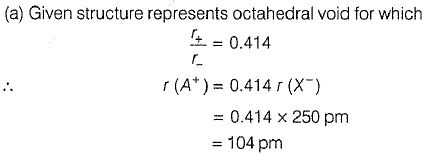QUESTION: 3

###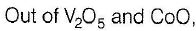Solution: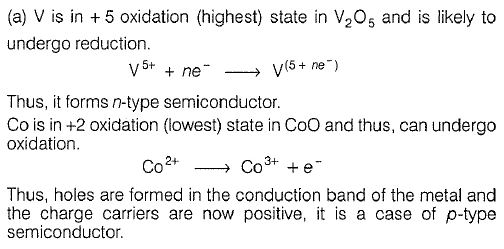QUESTION: 4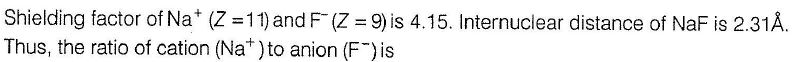Solution: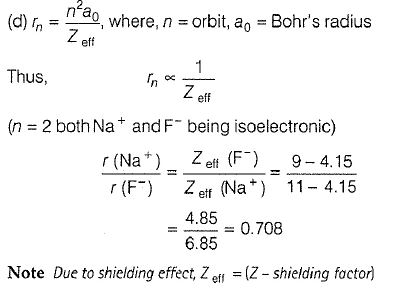QUESTION: 5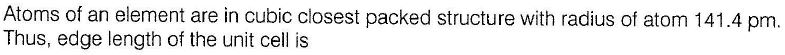Solution: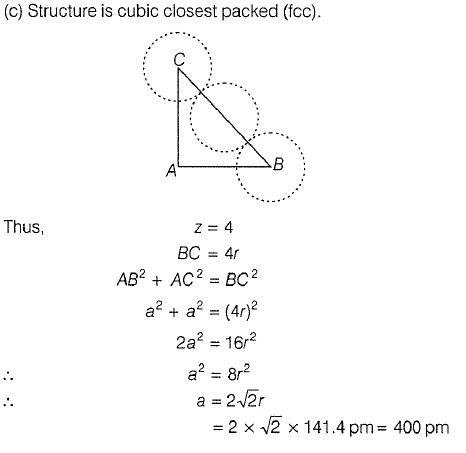QUESTION: 6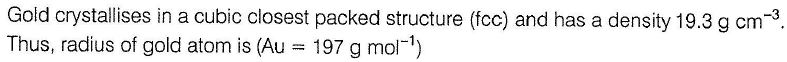Solution: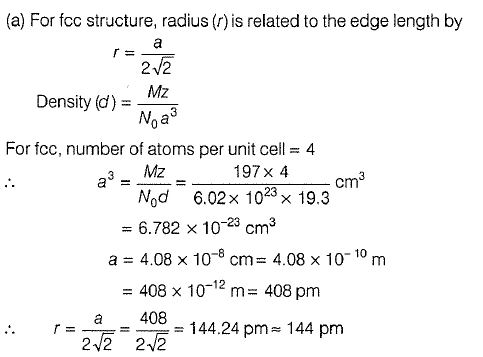QUESTION: 7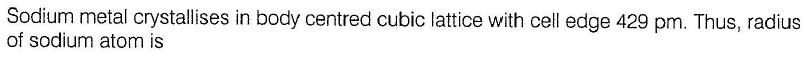Solution: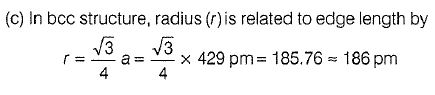QUESTION: 8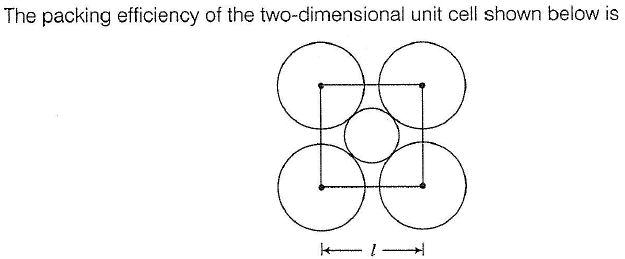Solution: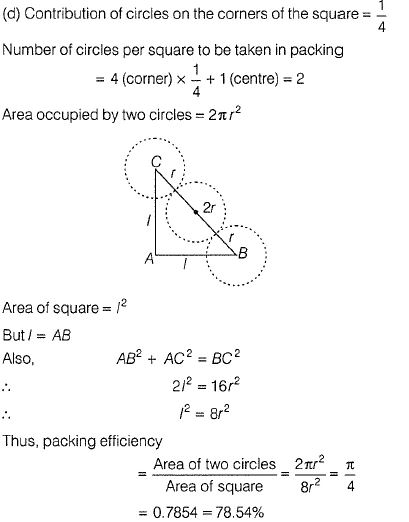QUESTION: 9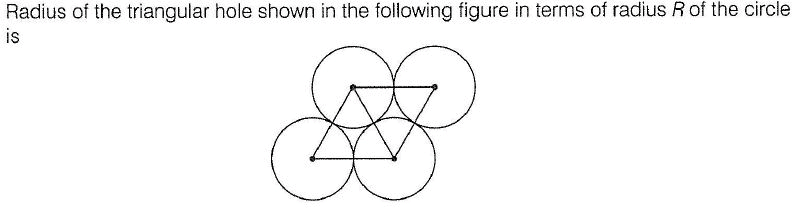Solution: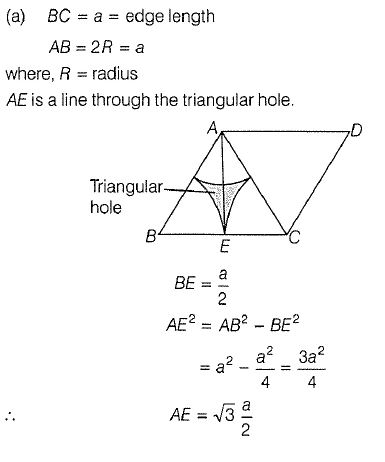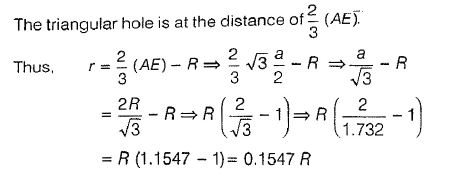QUESTION: 10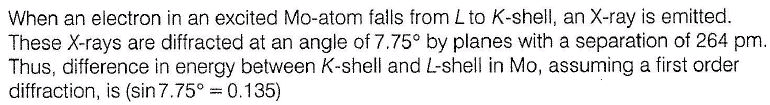Solution: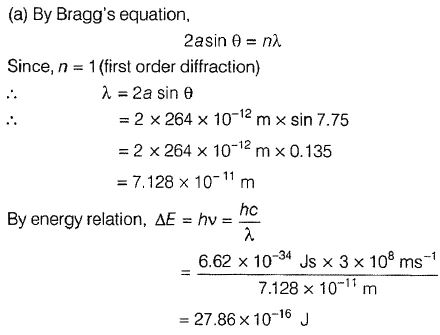QUESTION: 11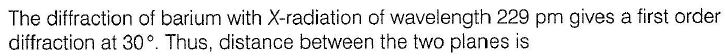Solution: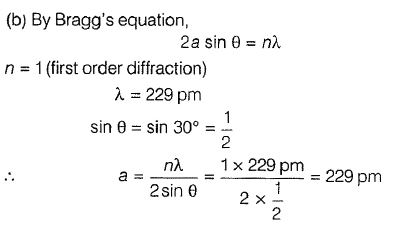QUESTION: 12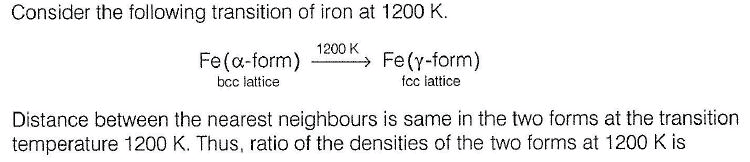Solution: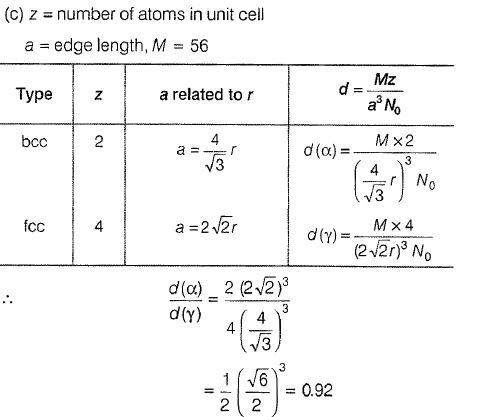QUESTION: 13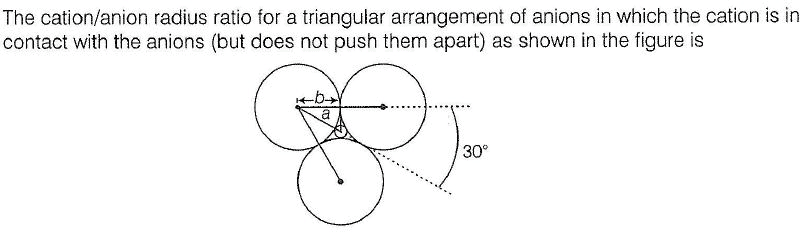Solution: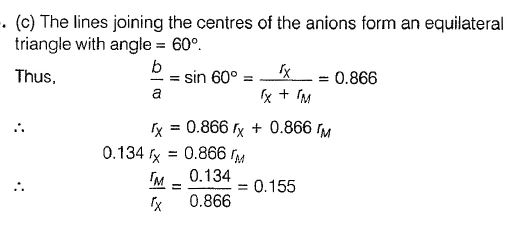QUESTION: 14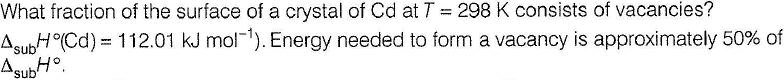Solution: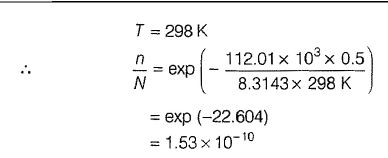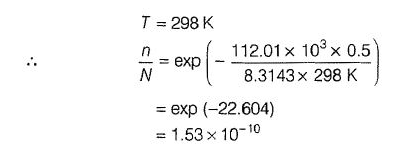QUESTION: 15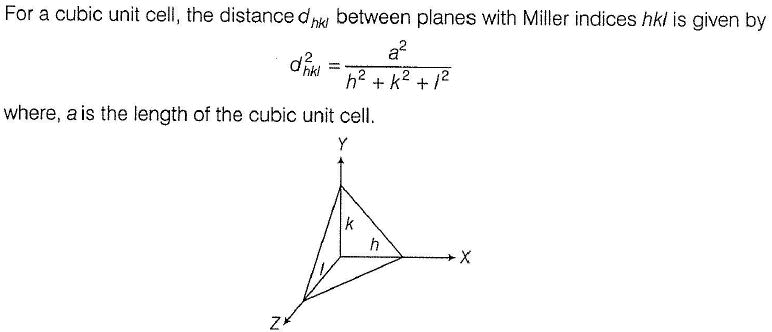Solution: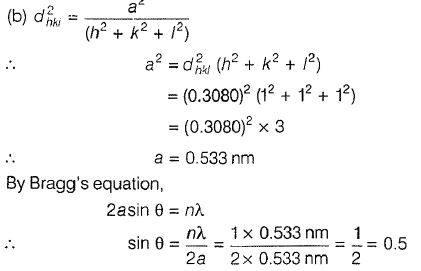QUESTION: 16

One or More than One Options Correct Type
This section contains 3 multiple type questions. Each question has 4 choices (a), (b), (c) and (d), out of which ONE or MORE THAN ONE are correct.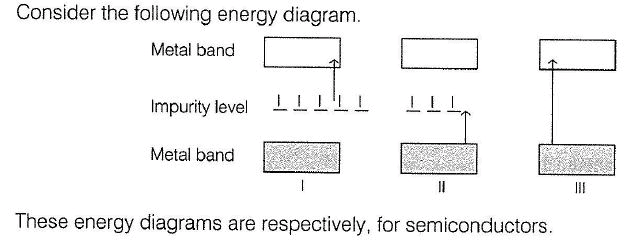Solution: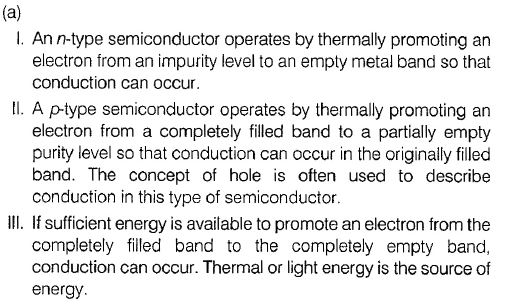QUESTION: 17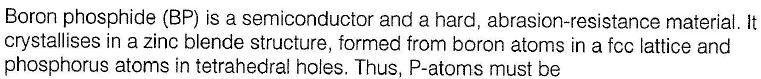Solution: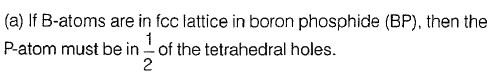*Multiple options can be correct
QUESTION: 18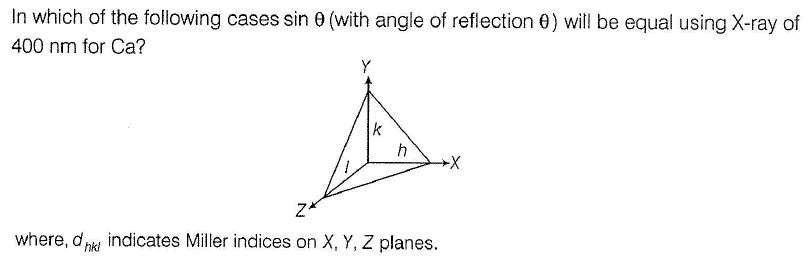Solution: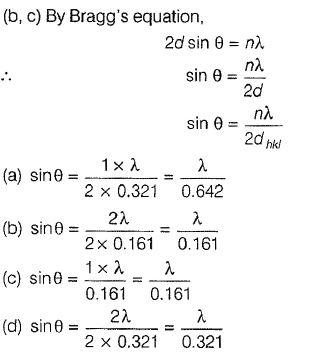QUESTION: 19

Matching List Type
Choices for the correct combination of elements from Column I and Column II are given as options (a), (b), (c) and (d), out of which one is correct.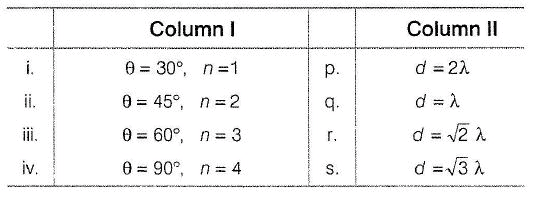Solution: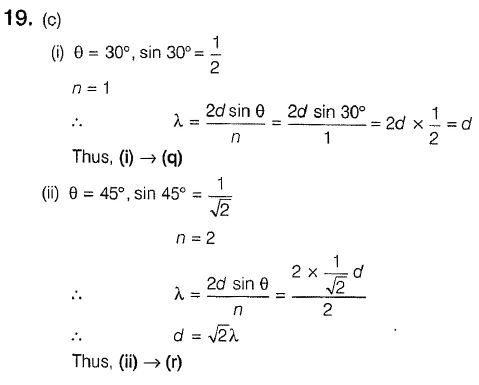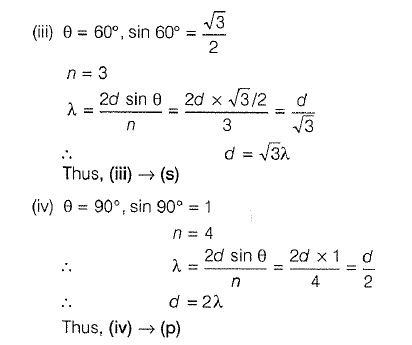QUESTION: 20

Comprehension Type
This section contains a passage describing theory, experiments, data, etc. Two questions related to the paragraph have been given. Each question has only one correct answer out of the given 4 options (a), (b), (c) and (d).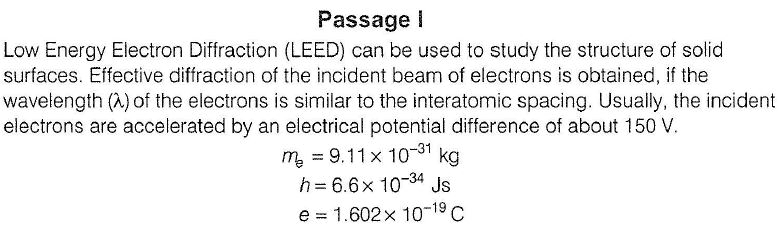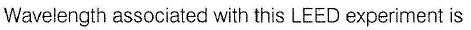Solution: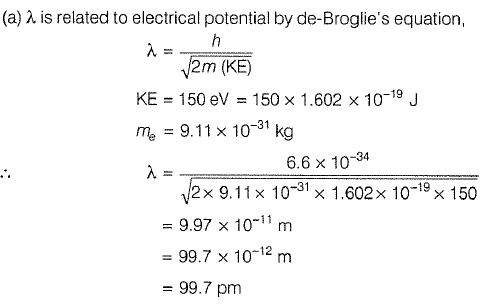QUESTION: 21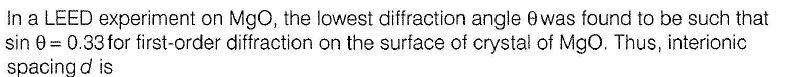Solution: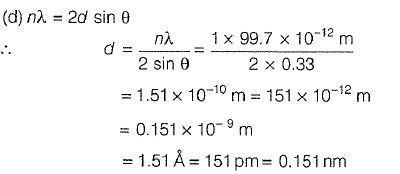QUESTION: 22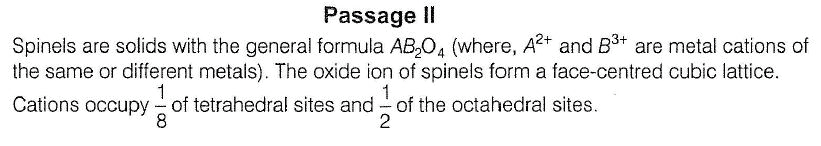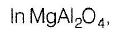Solution:

(c)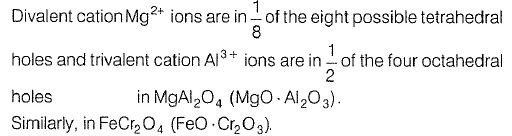QUESTION: 23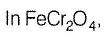Solution:

(a)QUESTION: 24

Passage III

Density of crystalline CsCl ( 168.56 g mol-1) is 4.0 g/ cm3. Volume occupied by a single CsCl ion pair in the crystal is

Solution:

(a)

QUESTION: 25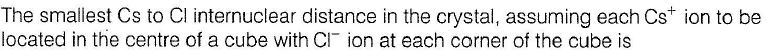Solution:

(a)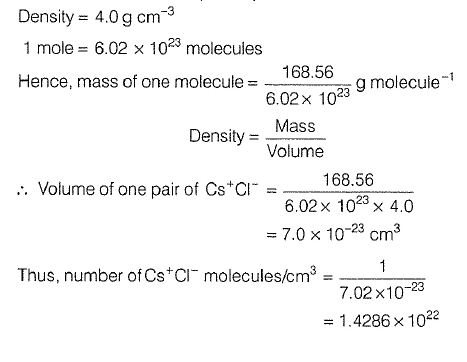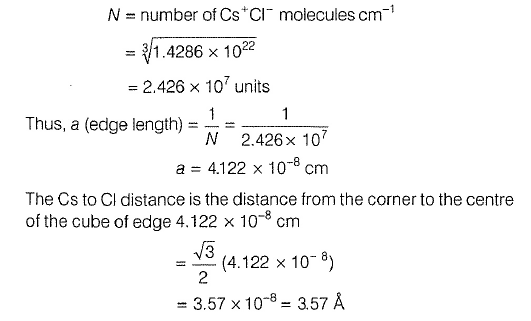*Answer can only contain numeric values
QUESTION: 26

One Integer Value Correct Type
This section contains 8 questions, when worked out will result in an integer value from 0 to 9 (both inclusive)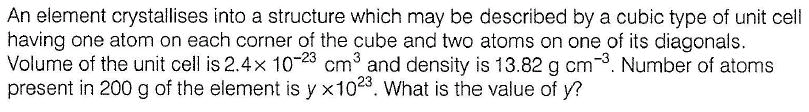Solution: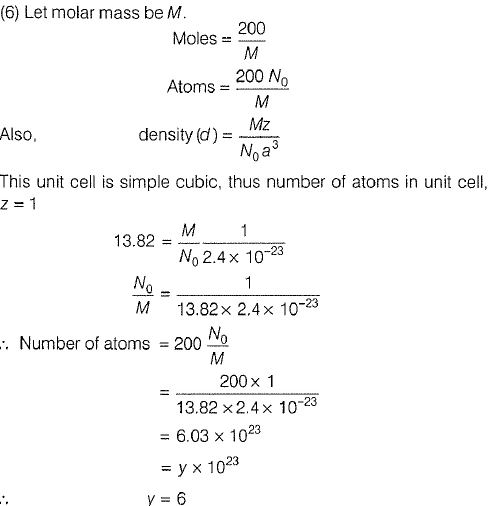*Answer can only contain numeric values
QUESTION: 27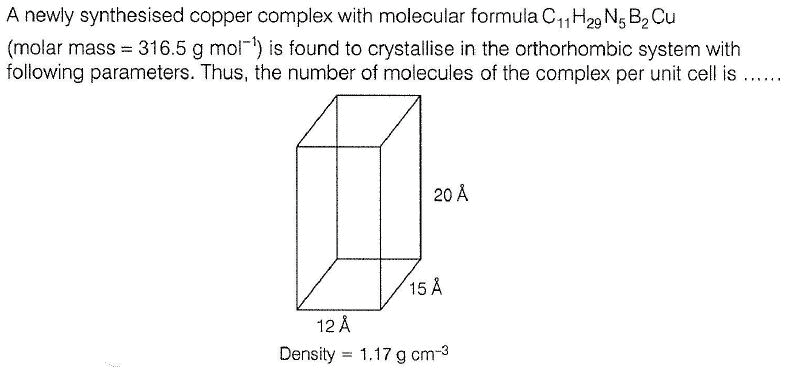Solution: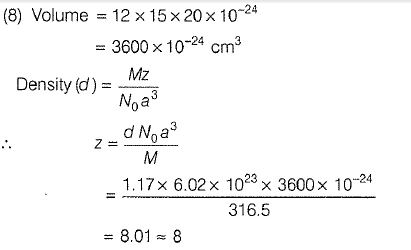*Answer can only contain numeric values
QUESTION: 28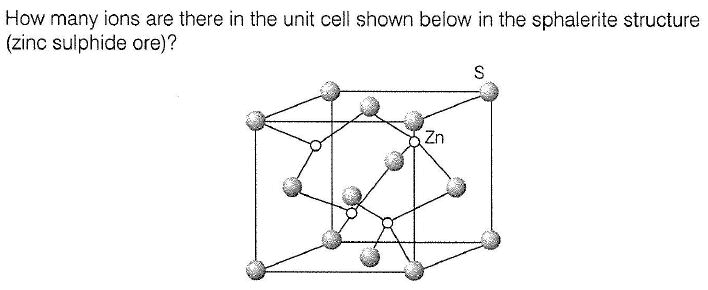Solution: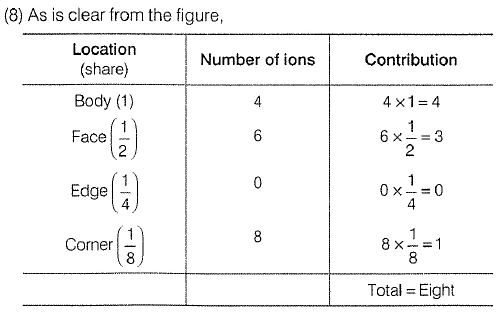*Answer can only contain numeric values
QUESTION: 29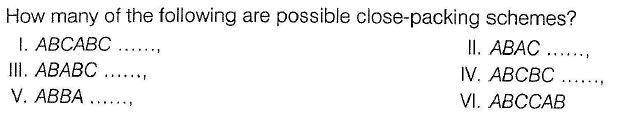Solution: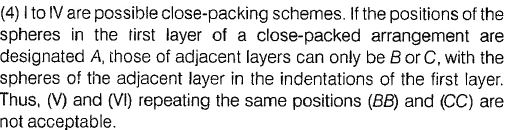*Answer can only contain numeric values
QUESTION: 30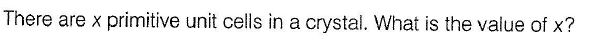Solution: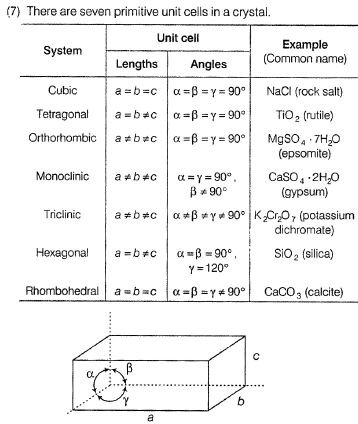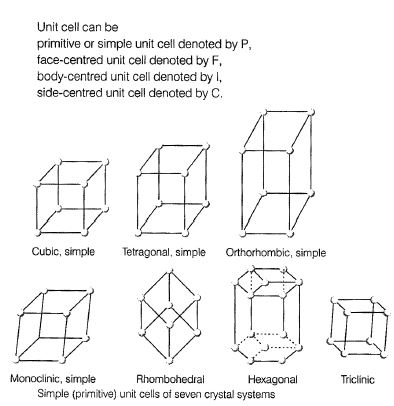*Answer can only contain numeric values
QUESTION: 31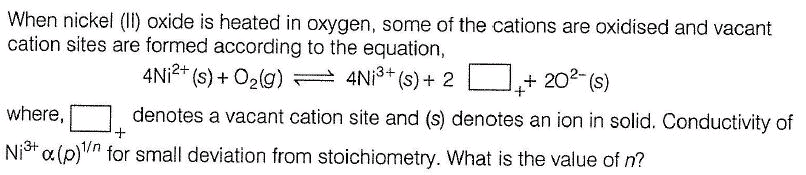Solution: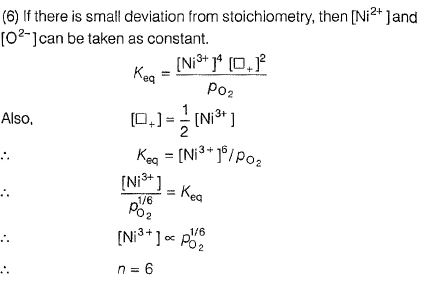*Answer can only contain numeric values
QUESTION: 32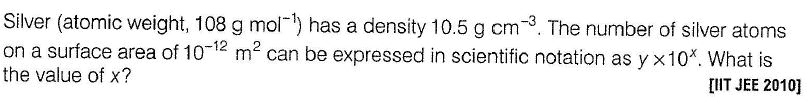Solution: